Deepak Scored 45->99%ile with Bounce Back Crack Course. You can do it too!

# Check whether the first polynomial is a factor of the second polynomial by applying the division algorithm :

Question:

Check whether the first polynomial is a factor of the second polynomial by applying the division algorithm :

(i) $g(t)=t^{2}-3, f(t)=2 t^{4}+3 t^{3}-2 t^{2}-9 t-12$

(ii) $g(x)=x^{3}-3 x+1, f(x)=x^{5}-4 x^{3}+x^{2}+3 x+1$

(iii) $g(x)=2 x^{2}-x+3, f(x)=6 x^{5}-x^{4}+4 x^{3}-5 x^{2}-x-15$

Solution:

$(i)$. Given $g(t)=t^{2}-3$

$f(t)=2 t^{4}+3 t^{3}-2 t^{2}-9 t-12$

Here, degree $(f(t))=4$ and

Degree $(g(t))=2$

Therefore, quotient $q(t)$ is of degree $4-2=2$

Remainder $r(t)$ is of degree 1 or less

Let $q(t)=a t^{2}+b t+c$ and

$r(t)=p t+q$

Using division algorithm, we have

$f(t)=g(t)+q(t)+r(t)$

$2 t^{4}+3 t^{3}-2 t^{2}-9 t-12=\left(a t^{2}+b t+c\right)\left(t^{2}-3\right)+p t+q$

$2 t^{4}+3 t^{3}-2 t^{2}-9 t-12=a t^{4}+b t^{3}+c t^{2}-3 a t^{2}-3 b t-3 c+p t+q$

$2 t^{4}+3 t^{3}-2 t^{2}-9 t-12=a t^{4}+b t^{3}-t^{2}(3 a-c)-t(3 b-p)-3 c+q$

Equating co-efficient of various powers of $t$, we get

On equating the co-efficient of $t^{4}$

$2 t^{4}=a t^{4}$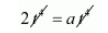$2=a$

On equating the co-efficient of $t^{3}$

$3 t^{3}=b t^{3}$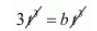$3=b$

On equating the co-efficient of $t^{2}$

$2=3 a-c$

$3=b$

On equating the co-efficient of $t^{2}$

$2=3 a-c$

Substituting $a=2$, we get

$2=3 \times 2-c$

$2=6-c$

$2-6=-c$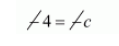$c=4$

On equating the co-efficient of $t$

$9=3 b-p$

Substituting $b=3$, we get

$9=9-p$

$9-9=-p$

$0=-p$

$p=0$

On equating constant term

$-12=-3 c+q$

Substituting $c=4$, we get

$-12=3 \times 4+q$

$-12=-12+q$

$-12+12=+q$

$0=q$

Quotient $q(t)=a t^{2}+b t+c$

$=2 t^{2}+3 t+4$

Remainder $r(t)=p t+q$

$=0 t+0$

$=0$

Clearly, $r(t)=0$

Hence, $g(t)$ is a factor of $f(t)$.

(ii) Given

$f(x)=x^{5}-4 x^{3}+x^{2}+3 x+1$

$g(x)=x^{3}-3 x+1$

Here, Degree $(f(x))=5$ and

Degree $(g(x))=3$

Therefore, quotient $q(x)$ is of degree $5-3=2$

Remainder $r(x)$ is of degree1

Let $q(x)=a x^{2}+b x+c$ and

$r(x)=p x+q$

Using division algorithm, we have

$f(x)=g(x) \times q(x)+r(x)$$x^{5}-4 x^{3}+x^{2}+3 x+1=\left(x^{3}-3 x+1\right)\left(a x^{2}+b x+c\right)+p x+q$

$x^{5}-4 x^{3}+x^{2}+3 x+1=\left(x^{3}-3 x+1\right)\left(a x^{2}+b x+c\right)+p x+q$

$x^{5}-4 x^{3}+x^{2}+3 x+1=a x^{5}-3 a x^{3}+a x^{2}+b x^{4}-3 b x^{2}+b x+c x^{3}-3 x c+c+p x+q$

$x^{5}-4 x^{3}+x^{2}+3 x+1=a x^{5}+b x^{4}-3 a x^{3}+c x^{3}+a x^{2}-3 b x^{2}+b x-3 x c+p x+c+q$

$x^{5}-4 x^{3}+x^{2}+3 x+1=a x^{5}+b x^{4}-x^{3}(3 a-c)+x^{2}(a-3 b)+x(b-3 c+p)+c+q$

Equating the co-efficient of various powers of $x$ on both sides, we get

On equating the co-efficient of $x^{5}$

$x^{5}=a x^{5}$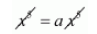$1=a$

On equating the co-efficient of $x^{4}$

$b x^{4}=0$

$b=\frac{0}{x^{4}}$

$b=0$

On equating the co-efficient of $x^{3}$

$3 a-c=4$

Substituting $a=1$ we get

$3 \times 1-c=4$

$3-c=4$

$-c=4-3$

$-c=1$

$c=-1$

On equating the co-efficient of $x$

$b-3 c+p=3$

Substituting $b=0$ and $c=-1$, we get

$0-3 \times-1+p=3$

$3+p=3$

$+p=3-3$

$p=0$

On equating constant term, we get

$c+q=1$

Substituting $c=-1$, we get

$-1+q=1$

$q=1+1$

$q=2$

Therefore, quotient $q(x)=a x^{2}+b x+c$

$=1 x^{2}+0 x-1$

$=x^{2}-1$

Remainder $r(x)=p x+q$

$=0 \times x+2$

$=2$

Clearly, $r(x)=2$

Hence, $g(x)$ is not a factor of $f(x)$.

(iii) Given,

$f(x)=6 x^{5}-x^{4}+4 x^{3}-5 x^{2}-x-15$

$g(x)=2 x^{2}-x+3$

Here, Degree $(f(x))=5$ and

Degree $(g(x))=2$

Therefore, quotient $q(x)$ is of degree $5-2=3$ and

Remainder $r(x)$ is of degree less than 1

Let $q(x)=a x^{3}+b x^{2}+c x+d$ and

$r(x)=p x+q$

Using division algorithm, we have

$f(x)=g(x) \times q(x)+r(x)$

$6 x^{5}-x^{4}+4 x^{3}-5 x^{2}-x-15=\left(2 x^{2}-x+3\right)\left(a x^{3}+b x^{2}+c x+d\right)+p x+q$

$6 x^{5}-x^{4}+4 x^{3}-5 x^{2}-x-15=2 a x^{5}-a x^{4}+3 a x^{3}+2 b x^{4}-b x^{3}+3 b x^{2}+2 c x^{3}-c x^{2}$

$6 x^{5}-x^{4}+4 x^{3}-5 x^{2}-x-15=2 a x^{5}-a x^{4}+2 b x^{4}+3 a x^{3}-b x^{3}+2 c x^{3}+3 b x^{2}+2 x^{2} d$

$-c x^{2}+3 c x-d x+p x+3 d+q$

$6 x^{5}-x^{4}+4 x^{3}-5 x^{2}-x-15=2 a x^{5}-x^{4}(a-2 b)+x^{3}(3 a-b+2 c)-x^{2}(c-3 b-2 d)$

$-x(d-3 c-p)+3 d+q$

Equating the co-efficient of various powers of $x$ on both sides, we get On equating the co-efficient of $x^{5}$

$2 a x^{5}=6 x^{5}$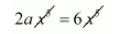$a=\frac{6}{2}$

$a=3$

On equating the co-efficient of $x^{4}$

$a-2 b=1$

Substituting $a=3$, we get

$3-2 b=1$

$-2 b=1-3$

$-b=\frac{-2}{-2}$$b=1$

On equating the co-efficient of $x^{3}$

$3 a-b+2 c=4$

Substituting $a=3$ and $b=1$, we get

$3 \times 3-1+2 c=4$

$9-1+2 c=4$

$8+2 c=4$

$2 c=4-8$

$2 c=-4$

$c=\frac{-4}{2}$

$c=-2$

On equating the co-efficient of $x^{2}$

$c-3 b-2 d=5$

Substituting $c=-2, b=1$, we get

$-2-3 \times 1-2 d=5$

$-2-3-2 d=5$

$-5-2 d=5$

$-2 d=5+5$

$-2 d=10$

$d=\frac{10}{-2}$

$d=-5$

On equating the co-efficient of $x$

$-3 c+d-p=1$

Substituting $c=-2$ and $d=-5$, we get

$-3 \times-2-5-p=1$

$6-5-p=1$

$1-p=1$

$-p=1-1$

$-p=0$

$0=p$

On equating constant term

$3 d+q=-15$

Substituting $d=-5$, we get

$3 \times-5+q=-15$

$-15+q=-15$

$q=-15+15$

$q=0$

Therefore, Quotient $q(x)=a x^{3}+b x^{2}+c x+d$

$=3 x^{3}+1 x^{2}-2 x-5$

Remainder $r(x)=p x+q$

$=0 x+0$

$=0$

Clearly, $r(x)=0$

Hence, $g(x)$ is a factor of $f(x)$.